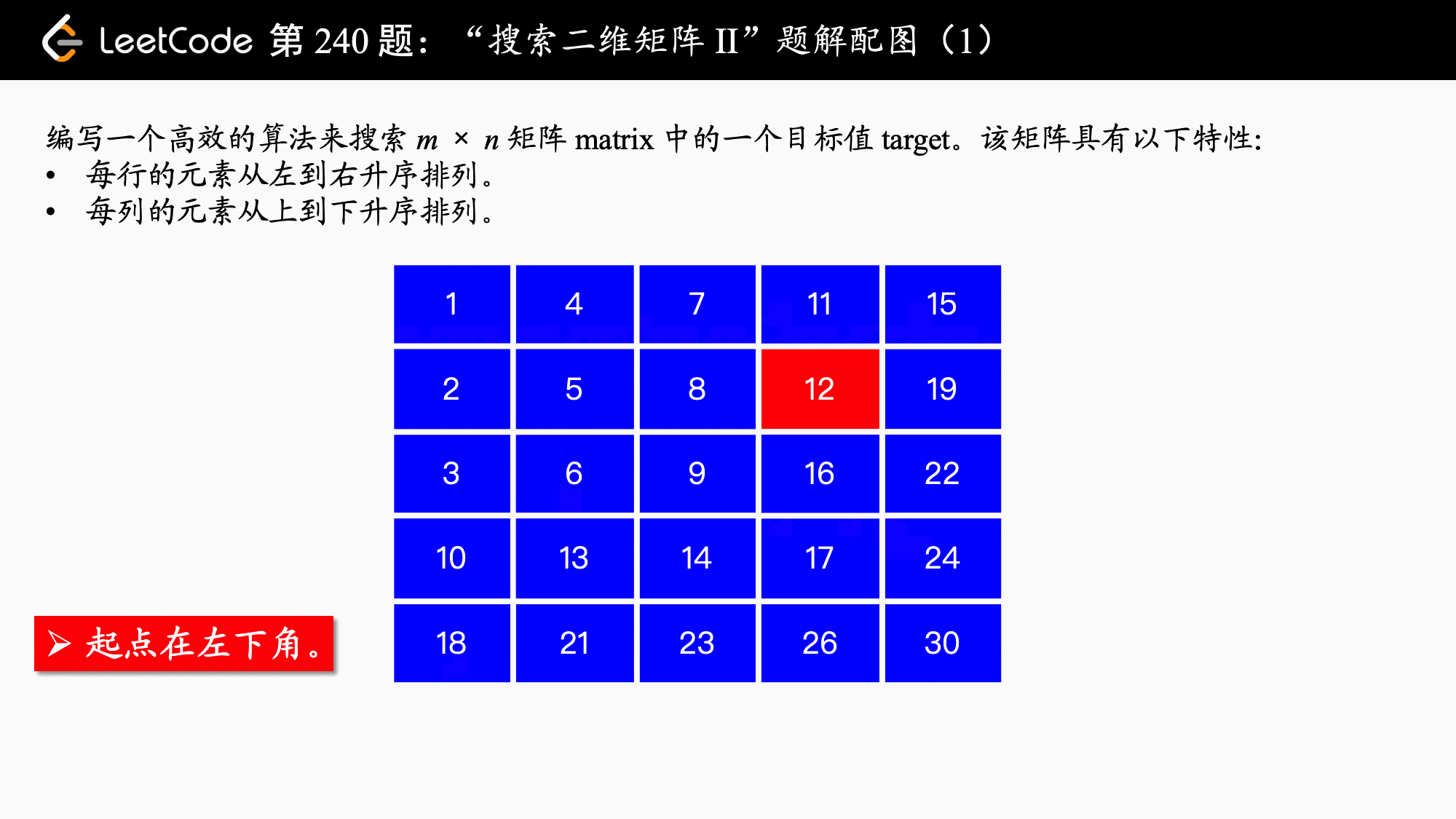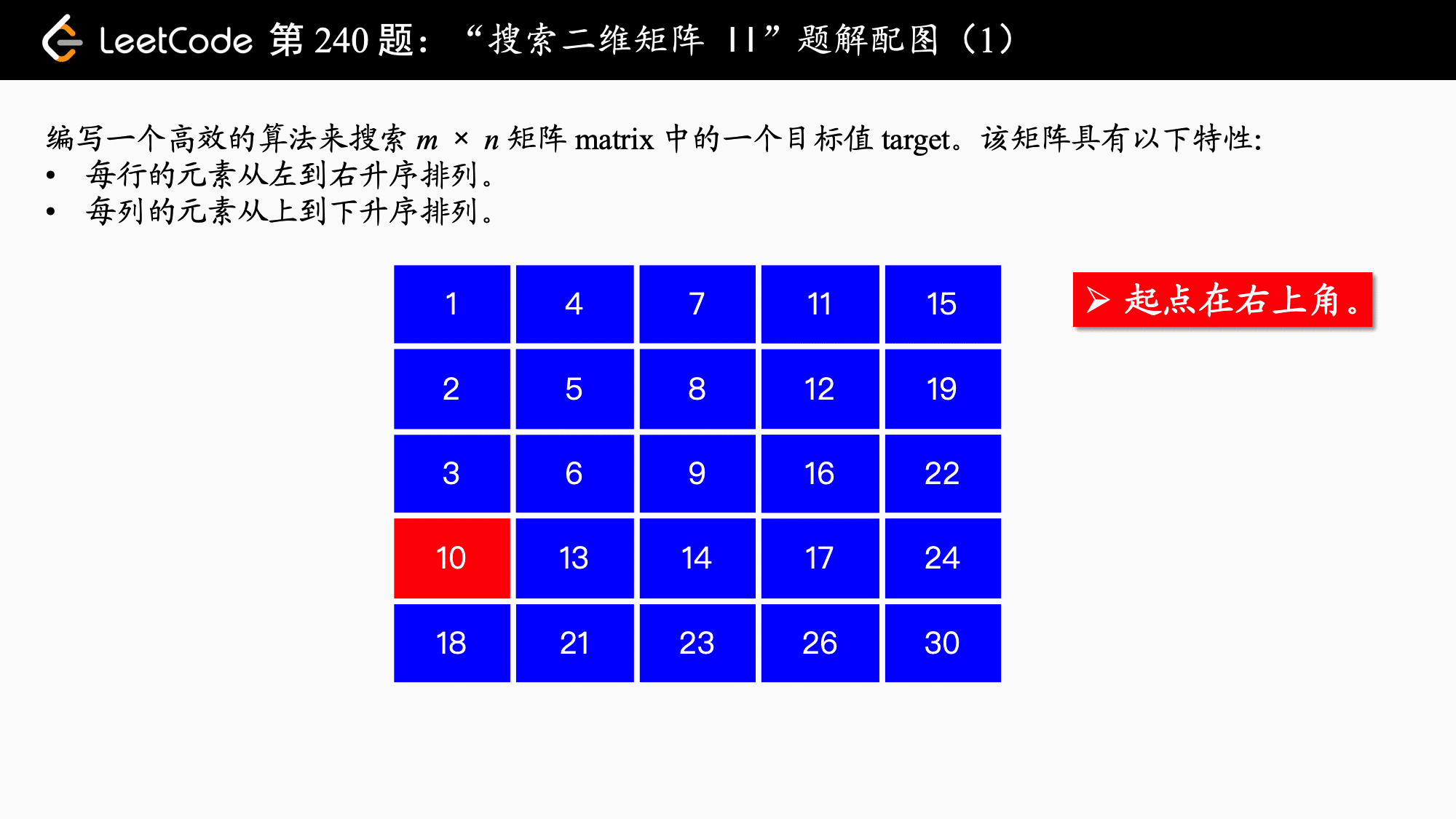# 「力扣」第 240 题：搜索二维矩阵 II

## 「力扣」第 240 题：搜索二维矩阵 II

[
[1, 4, 7, 11, 15],
[2, 5, 8, 12, 19],
[3, 6, 9, 16, 22],
[10, 13, 14, 17, 24],
[18, 21, 23, 26, 30]
]

## 排除法（不是什么新方法，就是你们最常看到的那个解法，从右下角、左上角开始）（Python 代码、Java 代码）

• 如果我们要用二分查找法，可以发现，如果一行的开头那个元素就比目标元素大，那么这一行的所有元素，以及行号大于这一行的元素都不在考虑的范围内。

• 我们首先尝试从左上角开始走，发现横着走数值增大，竖着走数值也增大，目标数值这在两个方向上都有可能存在。那如果我们从右上角或者左下角除法，找目标元素，那就不一样了，于是有了下面的“排除法”。

### 方法：排除法

1、如果选择左下角为起点Python 代码：

class Solution:
def searchMatrix(self, matrix, target):
# 特判
rows = len(matrix)
if rows == 0:
return False

cols = len(matrix)
if cols == 0:
return False

# 起点：左下角
x = rows - 1
y = 0
# 不越界的条件是：行大于等于 0，列小于等于 cols - 1

while x >= 0 and y < cols:
if matrix[x][y] > target:
x -= 1
elif matrix[x][y] < target:
y += 1
else:
return True
return False

Java 代码：

public class Solution {

public boolean searchMatrix(int[][] matrix, int target) {
int rows = matrix.length;
if (rows == 0) {
return false;
}
int cols = matrix.length;
if (cols == 0) {
return false;
}

// 起点：左下角
int x = rows - 1;
int y = 0;
// 不越界的条件是：行大于等于 0，列小于等于 cols - 1
while (x >= 0 && y < cols) {
// 打开注释，可以用于调试的代码
// System.out.println("沿途走过的数字：" + matrix[x][y]);
if (matrix[x][y] > target) {
x--;
} else if (matrix[x][y] < target) {
y++;
} else {
return true;
}
}
return false;
}

public static void main(String[] args) {
int[][] matrix = {
{1, 4, 7, 11, 15},
{2, 5, 8, 12, 19},
{3, 6, 9, 16, 22},
{10, 13, 14, 17, 24},
{18, 21, 23, 26, 30}
};
int target = 12;
Solution solution = new Solution();
boolean searchMatrix = solution3.searchMatrix(matrix, target);
System.out.println(searchMatrix);
}
}

• 时间复杂度：$O(M + N)$，$M$ 是这个矩阵的行数，$N$ 是这个矩阵的列数，我们看到，这种算法是“不回头”的，至多走 $M + N$ 步就能搜索到目标数值，或者判定目标数值在矩阵中不存子啊。
• 空间复杂度：$O(1)，算法使用了常数个变量$。

2、如果选择右上角为起点class Solution:
def searchMatrix(self, matrix, target):
# 特判
rows = len(matrix)
if rows == 0:
return False

cols = len(matrix)
if cols == 0:
return False

# 起点：右上
x = 0
y = cols -1

# 不越界的条件是：行小于等于 rows - 1，列大于等于 0
while x < rows and y >= 0:
if matrix[x][y] > target:
y -= 1
elif matrix[x][y] < target:
x += 1
else:
return True
return False
public class Solution {

public boolean searchMatrix(int[][] matrix, int target) {
// 特判
int rows = matrix.length;
if (rows == 0) {
return false;
}
int cols = matrix.length;
if (cols == 0) {
return false;
}

// 起点：右上角
int x = 0;
int y = cols - 1;

// 不越界的条件是：行小于等于 rows - 1，列大于等于 0
while (x < rows && y >= 0) {
// 打开注释，可以用于调试的代码
// System.out.println("沿途走过的数字：" + matrix[x][y]);
if (matrix[x][y] > target) {
y--;
} else if (matrix[x][y] < target) {
x++;
} else {
return true;
}
}
return false;
}

public static void main(String[] args) {
int[][] matrix = {
{1, 4, 7, 11, 15},
{2, 5, 8, 12, 19},
{3, 6, 9, 16, 22},
{10, 13, 14, 17, 24},
{18, 21, 23, 26, 30}
};
int target = 10;
Solution solution = new Solution();
boolean searchMatrix = solution2.searchMatrix(matrix, target);
System.out.println(searchMatrix);
}
}

（同上）。

上一篇「力扣」第 315 题：计算右侧小于当前元素的个数
「力扣」第 315 题：计算右侧小于当前元素的个数 题目地址 题解 LeetCode 第 315 题：计算右侧小于当前元素的个数 归并排序 + 索引数组（Python 代码、Java 代码） 归并排序（索引数组）如果有学习过一
2018-01-06「力扣」第 109 题：有序链表转换二叉搜索树
「力扣」第 109 题：有序链表转换二叉搜索树题解地址：分治法（Python 代码、Java 代码）。 说明：文本首发在力扣的题解版块，更新也会在第 1 时间在上面的网站中更新，这篇文章只是上面的文章的一个快照，您可以点击上面的链接看到其他
2018-01-04
目录